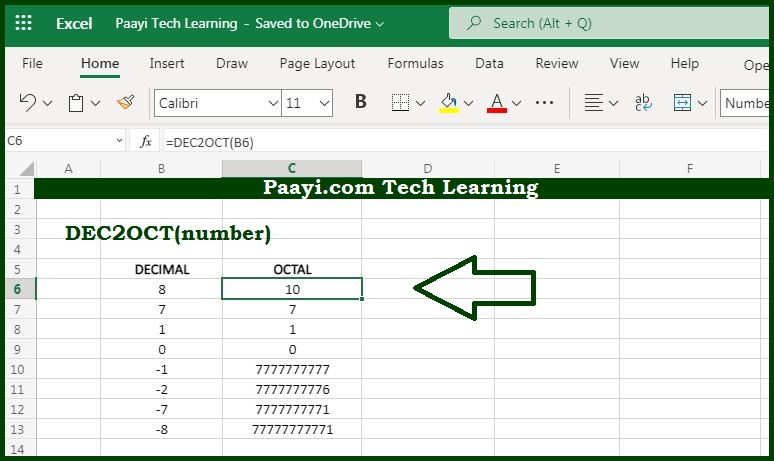# Learn How to Use Microsoft Excel DEC2OCT Function

Written by | 0 Comments | 625 Views

In this article, you will learn how to use the Microsoft Excel DEC2OCT function and its prime function in Microsoft Excel. You will also get to know the Microsoft Excel DEC2OCT function return value and syntax with the help of some examples.

Microsoft Excel DEC2OCT Function

The prime function of the Microsoft Excel DEC2OCT function is to convert decimal numbers to their octal equivalent. That means with the help of the DEC2OCT function you can able to convert a decimal number that represents its octal equivalent.

Return Value of DEC2OCT Function

The return value will be the octal number.

Syntax of DEC2OCT Function

=DEC2OCT(number, [places])

Where the arguments:

• number: It is the decimal number that you want to convert into octal equivalent.
• places: Holds the resulting octal number with zeros up to the specified number of digits. In case omitted returns the least number of characters required to represent the number (optional).

## How to Use Microsoft Excel DEC2OCT Function?As we know that DEC2OCT function used to convert the specified decimal number to its octal counterpart. Just make sure that the input must be the valid decimal number within the range of -2^29 to 2^29 - 1.